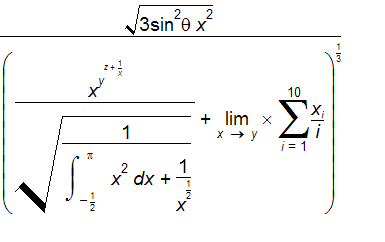﻿ Complex Equations

# Complex Equations

Top  Previous  Next
 The equation tool has only one limit on the complexity of the equations you can enter.   Matrices are limited to 20 by 20.     Because of the complexity of interpreting your entry, the equation tool will gradually slow as you enter a more complex equation but this will rarely impact on your use of the equation tool.In all likelihood, you will run out of steam before the equation tool does!  Please note that the equation above is NOT meant to make any sense. It is just there to show you how capable the equation tool is.